JEE  >  Test: Single Correct MCQs: Structure of Atom | JEE Advanced

# Test: Single Correct MCQs: Structure of Atom | JEE Advanced

Test Description

## 37 Questions MCQ Test Chemistry for JEE | Test: Single Correct MCQs: Structure of Atom | JEE Advanced

Test: Single Correct MCQs: Structure of Atom | JEE Advanced for JEE 2023 is part of Chemistry for JEE preparation. The Test: Single Correct MCQs: Structure of Atom | JEE Advanced questions and answers have been prepared according to the JEE exam syllabus.The Test: Single Correct MCQs: Structure of Atom | JEE Advanced MCQs are made for JEE 2023 Exam. Find important definitions, questions, notes, meanings, examples, exercises, MCQs and online tests for Test: Single Correct MCQs: Structure of Atom | JEE Advanced below.
Solutions of Test: Single Correct MCQs: Structure of Atom | JEE Advanced questions in English are available as part of our Chemistry for JEE for JEE & Test: Single Correct MCQs: Structure of Atom | JEE Advanced solutions in Hindi for Chemistry for JEE course. Download more important topics, notes, lectures and mock test series for JEE Exam by signing up for free. Attempt Test: Single Correct MCQs: Structure of Atom | JEE Advanced | 37 questions in 70 minutes | Mock test for JEE preparation | Free important questions MCQ to study Chemistry for JEE for JEE Exam | Download free PDF with solutions
 1 Crore+ students have signed up on EduRev. Have you?
Test: Single Correct MCQs: Structure of Atom | JEE Advanced - Question 1

### The number of neutrons in dipositive zinc ion with mass number 70 is (1979)

Detailed Solution for Test: Single Correct MCQs: Structure of Atom | JEE Advanced - Question 1

No. of neutrons = Mass number – Atomic number
= 70 – 30 = 40.

Test: Single Correct MCQs: Structure of Atom | JEE Advanced - Question 2

### Rutherford’s experiment on scattering of a-particles showed for the first time that the atom has (1981 - 1 Mark)

Detailed Solution for Test: Single Correct MCQs: Structure of Atom | JEE Advanced - Question 2

Rutherford’s scattering experiment led to the discovery of nucleus.

Test: Single Correct MCQs: Structure of Atom | JEE Advanced - Question 3

### Any p-orbital can accommodate upto (1983 - 1 Mark)

Detailed Solution for Test: Single Correct MCQs: Structure of Atom | JEE Advanced - Question 3

One p-orbital can accommodate up to two electrons with opposite spin while p-subshell can accommodate upto six electrons.

Test: Single Correct MCQs: Structure of Atom | JEE Advanced - Question 4

The principal quantum number of an atom is related to the (1983 - 1 Mark)

Detailed Solution for Test: Single Correct MCQs: Structure of Atom | JEE Advanced - Question 4

The principal quantum number (n) is related to the size of the orbital (n = 1, 2, 3.....)

Test: Single Correct MCQs: Structure of Atom | JEE Advanced - Question 5

Rutherford’s scattering experiment is related to the size of the (1983 - 1 Mark)

Detailed Solution for Test: Single Correct MCQs: Structure of Atom | JEE Advanced - Question 5

According to Rutherford's experiment. "The central part consisting of whole of the positive charge and most of the mass, called nucleus, is extremely small in size compared to the size of the atom."

Test: Single Correct MCQs: Structure of Atom | JEE Advanced - Question 6

The increasing order (lowest first) for the values of e/m (charge/mass) for electron (e), proton (p), neutron (n) and alpha particle (α) is : (1984 - 1 Mark)

Detailed Solution for Test: Single Correct MCQs: Structure of Atom | JEE Advanced - Question 6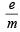for neutron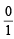= 0;  α-particle =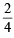= 0.5;

proton =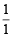= 1; electron =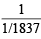= 1837

Test: Single Correct MCQs: Structure of Atom | JEE Advanced - Question 7

Correct set of four quantum numbers for the valence (outermost) electron of rubidium (Z = 37) is : (1984 - 1 Mark)

Detailed Solution for Test: Single Correct MCQs: Structure of Atom | JEE Advanced - Question 7

Rb has the configuration : 1s2 2s2p6 3s2p6d10 4s2p6 5s1; so n = 5, l = 0, m = 0 and s = +½ is correct set of quantum numbers for valence shell electron of Rb.

Test: Single Correct MCQs: Structure of Atom | JEE Advanced - Question 8

Which electronic level would allow the hydrogen atom to absorb a photon but not to emit a photon? (1984 - 1 Mark)

Detailed Solution for Test: Single Correct MCQs: Structure of Atom | JEE Advanced - Question 8

NOTE : Energy is emitted when electron falls from higher energy level to lower energy level and energy is absorbed when electron moves from lower level to higher level. 1s is the lowest energy level of electron in an atom.
∴ An electron in 1s level of hydrogen can absorb energy but cannot emit energy.

Test: Single Correct MCQs: Structure of Atom | JEE Advanced - Question 9

Bohr model can explain : (1985 - 1 Mark)

Detailed Solution for Test: Single Correct MCQs: Structure of Atom | JEE Advanced - Question 9

Bohr model can explain spectrum of atoms/ions containing one electron only.

Test: Single Correct MCQs: Structure of Atom | JEE Advanced - Question 10

The radius of an atomic nucleus is of the order of : (1985 - 1 Mark)

Detailed Solution for Test: Single Correct MCQs: Structure of Atom | JEE Advanced - Question 10

The radius of nucleus is of the order of 1.5 × 10–13 to 6.5 × 10–13 cm or 1.5 to 6.5 Fermi (1 Fermi = 10–13 cm)

Test: Single Correct MCQs: Structure of Atom | JEE Advanced - Question 11

Electromagnetic radiation with maximum wavelength is : (1985 - 1 Mark)

Detailed Solution for Test: Single Correct MCQs: Structure of Atom | JEE Advanced - Question 11

TIPS/Formulae : The following is the increasing order of wavelength or decreasing order of energy of electromagnetic radiations :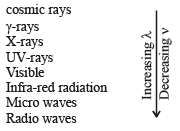Among given choices radiowaves have maximum wavelength.

Test: Single Correct MCQs: Structure of Atom | JEE Advanced - Question 12

Rutherford’s alpha particle scattering experiment eventually led to the conclusion that : (1986 - 1 Mark)

Detailed Solution for Test: Single Correct MCQs: Structure of Atom | JEE Advanced - Question 12

Electrons in an atom occupy the extra nuclear region.

Test: Single Correct MCQs: Structure of Atom | JEE Advanced - Question 13

Which on e of th e following sets of quantum numbers represents an impossible arrangement? (1986 - 1 Mark)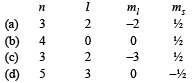Detailed Solution for Test: Single Correct MCQs: Structure of Atom | JEE Advanced - Question 13

If l = 2, m ≠ –3, m will vary from –2 to +2. i.e. possible values of m are –2, –1, 0, + 1 and + 2.

Test: Single Correct MCQs: Structure of Atom | JEE Advanced - Question 14

The ratio of the energy of a photon of 2000Å wavelength radiation to that of 4000Å radiation is : (1986 - 1 Mark)

Detailed Solution for Test: Single Correct MCQs: Structure of Atom | JEE Advanced - Question 14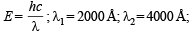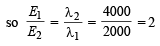Test: Single Correct MCQs: Structure of Atom | JEE Advanced - Question 15

The triad of nuclei that is isotonic is (1988 - 1 Mark)

Detailed Solution for Test: Single Correct MCQs: Structure of Atom | JEE Advanced - Question 15

Isotones have same number of neutrons. All atoms in triad (a) have same number of neutrons (= A – Z = 8).

Test: Single Correct MCQs: Structure of Atom | JEE Advanced - Question 16

The wavelength of a spectral line for an electronic transition is inversely related to : (1988 - 1 Mark)

Detailed Solution for Test: Single Correct MCQs: Structure of Atom | JEE Advanced - Question 16

Difference in the energy of the energy levels involved in the transition.

Test: Single Correct MCQs: Structure of Atom | JEE Advanced - Question 17

The orbital diagram in which the Aufbau principle is violated is : (1988 - 1 Mark)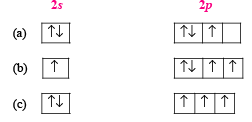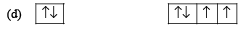Detailed Solution for Test: Single Correct MCQs: Structure of Atom | JEE Advanced - Question 17

According to Aufbau principle, the orbital of lower energy (2s) should be fully filled before the filling of orbital of higher energy starts.

Test: Single Correct MCQs: Structure of Atom | JEE Advanced - Question 18

The outermost electronic configuration of the most electronegative element is (1988 - 1 Mark)

Detailed Solution for Test: Single Correct MCQs: Structure of Atom | JEE Advanced - Question 18

TIPS/Formulae : The element having highest tendency to accept the electron will be most electronegative element.
Configuration ns2, np5 means it requires only one electron to attain nearest noble gas configuration. So, it will be most electronegative element among given choices.

Test: Single Correct MCQs: Structure of Atom | JEE Advanced - Question 19

The correct ground state electronic configuration of chromium atom is : (1989 - 1 Mark)

Detailed Solution for Test: Single Correct MCQs: Structure of Atom | JEE Advanced - Question 19

NOTE : Exactly half filled orbitals are more stable than nearly half filled orbitals.
Cr (At. no. 24) has configuration [Ar] 3d5, 4s1.

Test: Single Correct MCQs: Structure of Atom | JEE Advanced - Question 20

The correct set of quantum numbers for the unpaired electron of chlorine atom is : (1989 - 1 Mark)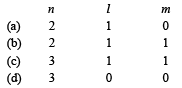Detailed Solution for Test: Single Correct MCQs: Structure of Atom | JEE Advanced - Question 20

Electronic configuration of chlorine is [Ne] 3s2, 3p5
∴ Unpaired electron is found in 3p sub-shell.
∴ n = 3, l = 1, m = 1

Test: Single Correct MCQs: Structure of Atom | JEE Advanced - Question 21

Which of the following does not characterise X-rays? (1992 - 1 Mark)

Detailed Solution for Test: Single Correct MCQs: Structure of Atom | JEE Advanced - Question 21

X-rays can ionise gases and cannot get deflected by electric and magnetic fields, wavelength of these rays is 150 to 0.1Å. Thus the wavelength of X-rays is shorter than that of u.v. rays.

Test: Single Correct MCQs: Structure of Atom | JEE Advanced - Question 22

Which of the following relates to photons both as wave motion and as a stream of particles? (1992 - 1 Mark)

Detailed Solution for Test: Single Correct MCQs: Structure of Atom | JEE Advanced - Question 22

As packet of energy equal to hv; as wave having frequency v.

Test: Single Correct MCQs: Structure of Atom | JEE Advanced - Question 23

A 3p orbital has : (1995S)

Detailed Solution for Test: Single Correct MCQs: Structure of Atom | JEE Advanced - Question 23

TIPS/Formulae : Total nodes = n – l
No. of radial nodes = n – l – 1
No. of angular nodes = l
For 3p sub-shell, n = 3, l = 1
∴ No. of radial nodes = n – l – 1 = 3 – 1 – 1 = 1
∴ No. of angular nodes = l = 1

Test: Single Correct MCQs: Structure of Atom | JEE Advanced - Question 24

The orbital angular momentum of an electron in 2s orbital is: (1996 - 1 Mark)

Detailed Solution for Test: Single Correct MCQs: Structure of Atom | JEE Advanced - Question 24

TIPS/Formulae : Orbital angular momentum (mvr) =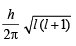For 2s orbital, l (azimuthal quantum number) = 0

∴ Orbital angular momentum = 0.

Test: Single Correct MCQs: Structure of Atom | JEE Advanced - Question 25

For a d-electron, the orbital angular momentum is (1997 - 1 Mark)

Detailed Solution for Test: Single Correct MCQs: Structure of Atom | JEE Advanced - Question 25

The expression for orbital angular momentum is Angular momentum =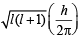For d orbital, l = 2.
Hence,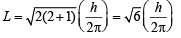Test: Single Correct MCQs: Structure of Atom | JEE Advanced - Question 26

The electron s, iden tified by quantum numbers n and l, (i) n = 4, l = 1, (ii) n = 4, l = 0, (iii) n = 3, l = 2, and (iv) n = 3, l = 1 can be placed in order of increasing energy, from the lowest to highest, as (1999 - 2 Marks)

Detailed Solution for Test: Single Correct MCQs: Structure of Atom | JEE Advanced - Question 26

TIPS/Formulae : The two guiding rules to arrange the various orbitals in the increasing energy are:
(i) Energy of an orbital increases with increase in the value of n + l.
(ii) Of orbitals having the same value of n + l, the orbital with lower value of n has lower energy.
Thus for the given orbitals, we have
(i)   n + l = 4 + 1 = 5
(ii)  n + l = 4 + 0 = 4
(iii) n + l = 3 + 2 = 5
(iv) n + l = 3 + 1 = 4
Hence, the order of increasing energy is (iv) < (ii) < (iii) < (i)

Test: Single Correct MCQs: Structure of Atom | JEE Advanced - Question 27

The number of nodal planes in a px orbital is (2000S)

Detailed Solution for Test: Single Correct MCQs: Structure of Atom | JEE Advanced - Question 27

px orbital being dumbell shaped, have number of nodal planes = 1, in yz plane.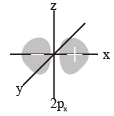Test: Single Correct MCQs: Structure of Atom | JEE Advanced - Question 28

The electronic configuration of an element is 1s2, 2s2 2p6, 3s2 3p6 3d5, 4s1. This represents its (2000S)

Detailed Solution for Test: Single Correct MCQs: Structure of Atom | JEE Advanced - Question 28

3d 54s1 system is more stable than 3d44s2, hence former is the ground state configuration.

Test: Single Correct MCQs: Structure of Atom | JEE Advanced - Question 29

The wavelength associated with a golf ball weighing 200 g and moving at a speed of 5 m/h is of the order (2001S)

Detailed Solution for Test: Single Correct MCQs: Structure of Atom | JEE Advanced - Question 29

TIPS/Formulae : According to de-Broglie’s equation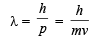Given, h = 6.6 × 10–34 Js, m = 200 × 10–3 kg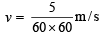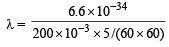= 2.38 × 10–30 m

Test: Single Correct MCQs: Structure of Atom | JEE Advanced - Question 30

The quantum numbers +1/2 and –1/2 for the electron spin represent (2001S)

Detailed Solution for Test: Single Correct MCQs: Structure of Atom | JEE Advanced - Question 30

The term spin implies that this magnetic moment is produced by the electron charge as the electron rotates about its own axis. Although this conveys a vivid mental picture of the source of the magnetism, the electron is not an extended body and its rotation is meaningless. Electron spin has no classical counterpart; the magnetic moment is a consequence of relativistic shifts in local space and time due to the high effective velocity of the electron in the atom.

Test: Single Correct MCQs: Structure of Atom | JEE Advanced - Question 31

Rurtherford's experiment, which established the nuclear model of the atom, used a beam of (2002S)

Detailed Solution for Test: Single Correct MCQs: Structure of Atom | JEE Advanced - Question 31

Rutherfor d's experiment was actually α -par ticle scattering experiment. α -Particle is doubly positively charged heliumion i.e., He - nucleus.

Test: Single Correct MCQs: Structure of Atom | JEE Advanced - Question 32

If the nitrogen atom has electronic configuration 1s7, it would have energy lower than that of the normal ground state configuration 1s22s22p3, because the electrons would be closer to the nucleus. Yet 1s7 is not observed because it violates.(2002S)

Detailed Solution for Test: Single Correct MCQs: Structure of Atom | JEE Advanced - Question 32

As per Pauli Exclusion Principle  "no two electrons in the same atom can have all the four quantum numbers equal or an orbital cannot contain more than two electrons and it can accommodate two electrons only when their directions of spins are opposite".

Test: Single Correct MCQs: Structure of Atom | JEE Advanced - Question 33

The radius of which of the following orbit is same as that of the first Bohr ’s orbit of hydrogen atom? (2004S)

Detailed Solution for Test: Single Correct MCQs: Structure of Atom | JEE Advanced - Question 33

TIPS/Formulae :​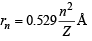For hydrogen, n = 1 and Z = 1 ; ∴ rH = 0.529

For Be3+, n = 2 and Z = 4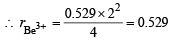Test: Single Correct MCQs: Structure of Atom | JEE Advanced - Question 34

The n umber of radial nodes of 3s and 2p orbitals are respectively (2005S)

Detailed Solution for Test: Single Correct MCQs: Structure of Atom | JEE Advanced - Question 34

TIPS/Formulae : Number of radial nodes = (n - l - 1)
For 3s: n = 3, l = 0 (Number of radial node = 2)
For 2p: n =2, l = 1 (Number of radial node = 0)

Test: Single Correct MCQs: Structure of Atom | JEE Advanced - Question 35

Given that the abundances of isotopes 54Fe, 56Fe and 57 Fe are 5%, 90% and 5%, respectively, the atomic mass of Fe is

Detailed Solution for Test: Single Correct MCQs: Structure of Atom | JEE Advanced - Question 35

Average atomic mass of Fe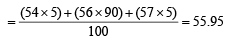Test: Single Correct MCQs: Structure of Atom | JEE Advanced - Question 36

The kinetic energy of an electron in the second Bohr orbit of a hydrogen atom is [a0 is Bohr radius] : (2012)

Detailed Solution for Test: Single Correct MCQs: Structure of Atom | JEE Advanced - Question 36

As per Bohr’s postulate,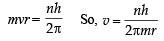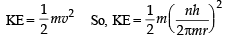Since,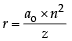So, for 2nd Bohr orbit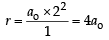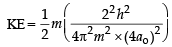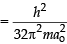Test: Single Correct MCQs: Structure of Atom | JEE Advanced - Question 37

P is the probability of finding the 1s electron of hydrogen atom in a spherical shell of infinitesimal thickness, dr, at a distance r from the nucleus. The volume of this shell is 4πr2dr. The qualitative sketch of the dependence of P on r is (JEE Adv. 2016)

Detailed Solution for Test: Single Correct MCQs: Structure of Atom | JEE Advanced - Question 37

Radial probability function curve for 1s is (D).
Here P is 4πr2R2.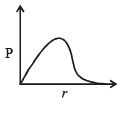## Chemistry for JEE

322 videos|586 docs|368 tests
 Use Code STAYHOME200 and get INR 200 additional OFF Use Coupon Code
Information about Test: Single Correct MCQs: Structure of Atom | JEE Advanced Page
In this test you can find the Exam questions for Test: Single Correct MCQs: Structure of Atom | JEE Advanced solved & explained in the simplest way possible. Besides giving Questions and answers for Test: Single Correct MCQs: Structure of Atom | JEE Advanced, EduRev gives you an ample number of Online tests for practice

## Chemistry for JEE

322 videos|586 docs|368 tests